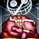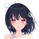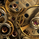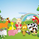4751 просмотров
Introduction

Forecasting is a blurry science that deal with lot of uncertainty. Most of the time forecasting is made with the assumption that past values can be used to forecast a time series, the accuracy of the forecast depend on the type of time series, the pre-processing applied to it, the forecast model and the parameters of the model.

In tradingview we don't have much forecasting models appart from the linear regression which is definitely not adapted to forecast financial markets, instead we mainly use it as support/resistance indicator. So i wanted to try making a forecasting tool based on the lsma that might provide something at least interesting, i hope you find an use to it.

The Method

Remember that the regression model and the lsma are closely related, both share the same equation ax + b but the lsma will use running parameters while a and b are constants in a linear regression , the last point of the lsma of period p is the last point of the linear regression that fit a line to the price at time p to 1, try to add a linear regression with count = 100 and an lsma of length = 100 and you will see, this is why the lsma is also called "end point moving average".

The forecast of the linear regression is the linear extrapolation of the fitted line, however the proposed indicator forecast is the linear extrapolation between the value of the lsma at time length and the last value of the lsma when short term extrapolation is false, when short term extrapolation is checked the forecast is the linear extrapolation between the lsma value prior to the last point and the last lsma value.

long term extrapolation, length = 1000

short term extrapolation, length = 1000

How To Use

Intervals are create from the running mean absolute error between the price and the lsma . Those intervals can be interpreted as possible support and resistance levels when using long term extrapolation, make sure that the intervals have been priorly tested, this mean the intervals are more significants.

The short term extrapolation is made with the assumption that the price will follow the last two lsma points direction, the forecast tend to become inaccurate during a trend change or when noise affect heavily the lsma .

You can test both method accuracy with the replay mode.

Comparison With The Linear Regression

Both methods share similitudes, but they have different results, lets compare them.

In blue the indicator and in red a linear regression of both period 200, the linear regression is always extremely conservative since she fit a line using the least squares method, at the contrary the indicator is less conservative which can be an advantage as well as a problem.

Conclusion

Linear models are good when what we want to forecast is approximately linear, thats not the case with market price and this is why other methods are used. But the use of the lsma to provide a forecast is still an interesting method that might require further studies.

« Je suis las des cruautés de mes semblables, qui ne sont pas mes pareils.

« Je prendrai l’essor et je m’envolerai vers la mer.

« Je connaîtrai le goût des brises du large. J’entendrai les grands cris de la tempête.

## КомментарииThis is some great food for thought, you got my rusty brain thinking now. Really good, loved your work on this and explanation. Thanks! Followed you on TV.
ОтветитьOwl
ОтветитьAgain, thank you.
Been waiting for this for a while.
Thank you, thank you, thank you,.......
Ответитьaaahopper
Ответитьalexgrover
@alexgrover, I tried to set up indicators with crossover(high,a) and crossunder(low,b).
Unfortunately, it did not work properly.
Would you be able to assist?
Thank you one million times.
Ответитьaaahopper
@aaahopper, The Indicator does not have numerical values, so its not possible todo much, i'am working on it.
ОтветитьAppreciated your generous sweet heart for sharing this wonderful stuff.
Very detail and nice explanation; keep it up bro and happy trading =D
ОтветитьICEKI
@ICEKI, I was afraid kinda of the explanation, i'am glad it turned out to be detailed, thanks for your feedback and support as always :)
Ответить